# Maths Calculator Skills You Didn’t Know Your Calculator Can Do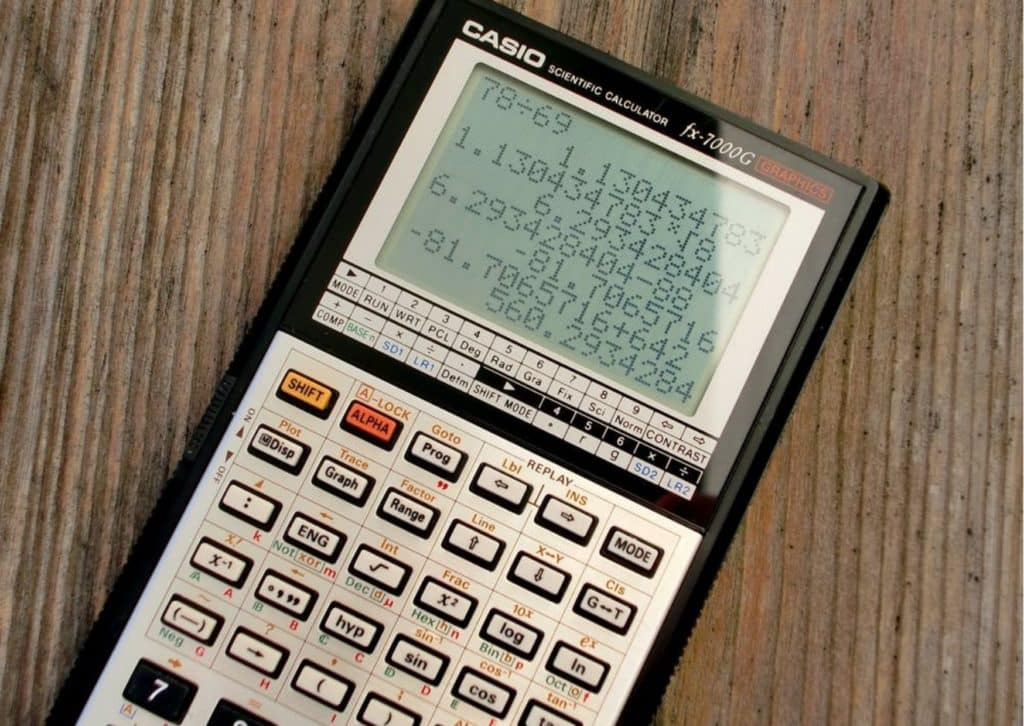Soon after they enter high school or secondary school, students are allowed to use calculators during their exams. It is imperative to understand the principles of mathematical calculation without the aid of a calculator, which is why elementary school children are not permitted to use a calculator during their math exams. In higher education, a calculator is essential because it enables students to complete more difficult calculations that are simple to complete on a calculator. Thus, we will examine some math calculator skills in this post to help pupils boost their game in arithmetic tests.

## Does using a calculator benefit students?

Researchers from the University of Nottingham and the UCL Institute of Education found no evidence that using calculators in class hurts students’ math ability. Instead, knowing how to use a calculator in math helps improve students’ math and calculation ability. Calculators can be a very useful tool for learning how to compute without a calculator. Students use calculators less frequently but more effectively as a result of improving their math skills and developing their ability to self-regulate.

## 7 Calculator Skills for Math

Here are a few arithmetic calculator abilities that, assuming you’re a high school student who uses one of the more sophisticated calculators, you may or may not already know but that will come in very handy when you’re taking math exams. You may need to cycle through some of your calculator’s accessible capabilities to find out, even if not all calculators offer the same features. Nevertheless, the following list of math calculator skills will come in handy for you during your arithmetic exams.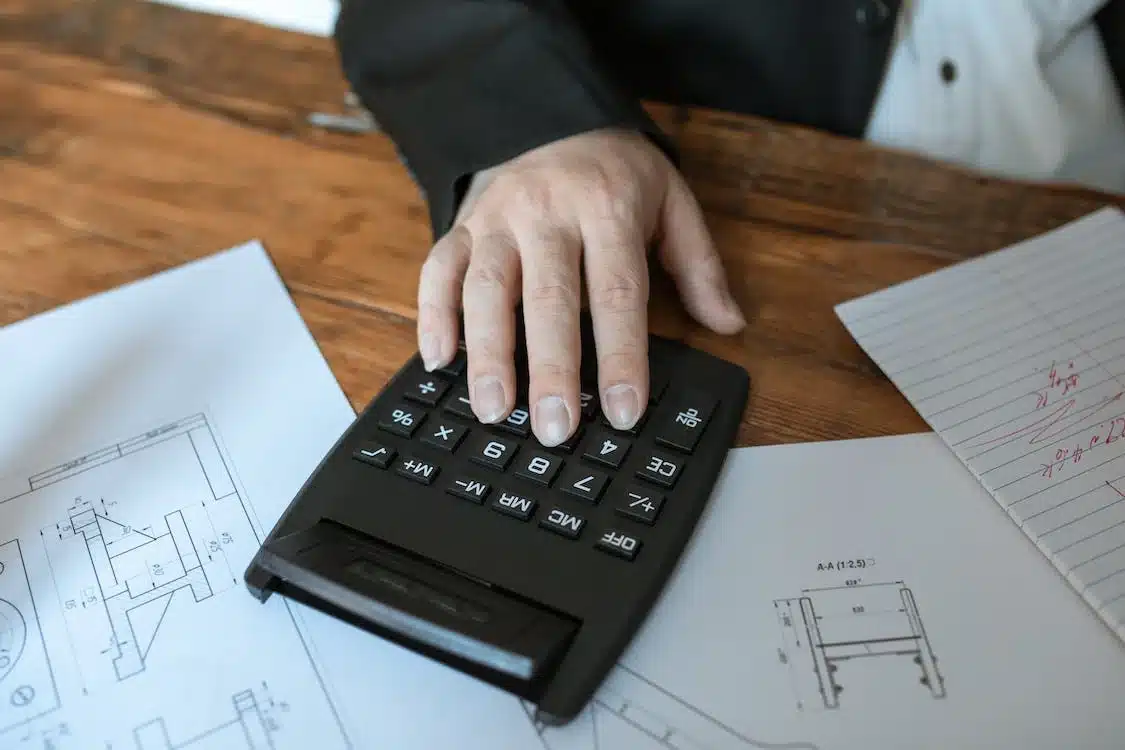1- Value Storage

One of the most fundamental tasks that every scientific calculator is capable of performing is this one. You can utilize previously saved numbers for optimal accuracy and avoid mistakes by storing them in your calculator. You can also benefit from double-checking your values in case you wrote them incorrectly on your exam scripts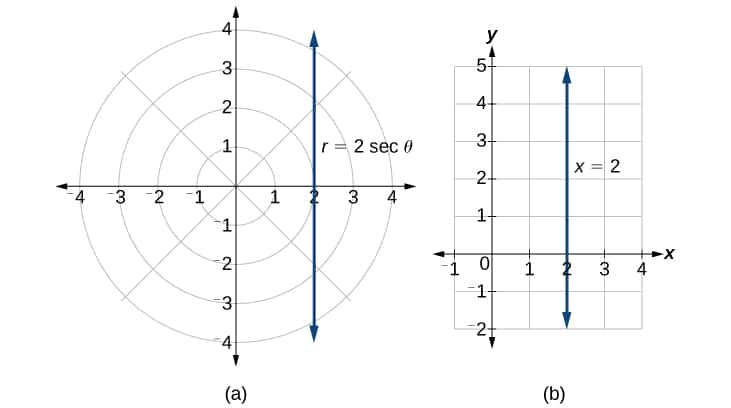2 – Changing the form from polar to rectangle

In high school, we studied a variety of subjects, including polar and rectangular forms. Despite being expressed in two distinct ways, they are coordinates. Although the process of changing from one to the other is not particularly laborious, it can be sped up with a calculator. The calculator can also be used to carry out the four operations. Multiplication and division can only be done in polar form, while addition and subtraction can only be done in rectangle form.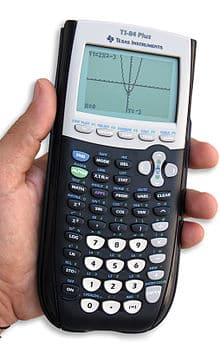3 – Making a graph in table mode

Some calculators may offer this function, albeit not all calculators may be compatible with it. In order to get tutorials on how to utilize table mode for graphing, search for your calculator model on YouTube. Yes, having this method allows you to avoid manually computing each point one at a time. You only need to enter the equation for the line and its points. The x coordinates you require to finish plotting your graph will then be available for retrieval.

4 – Calculating the mean, variance, and standard deviation using the statistics mode

These three crucial statistical terms will be familiar to high school students who study statistics. You will need to be aware of at least one or two of these three given distributions in order to perform any hypothesis. So, did you know that if you are given a distribution, you can calculate the mean, variance, and standard deviation? You might just be able to get some help with this from your calculator. If you provide a distribution of numbers, certain scientific calculators will calculate the mean, variance, and standard deviation. This can be used by students to confirm if they computed the values accurately.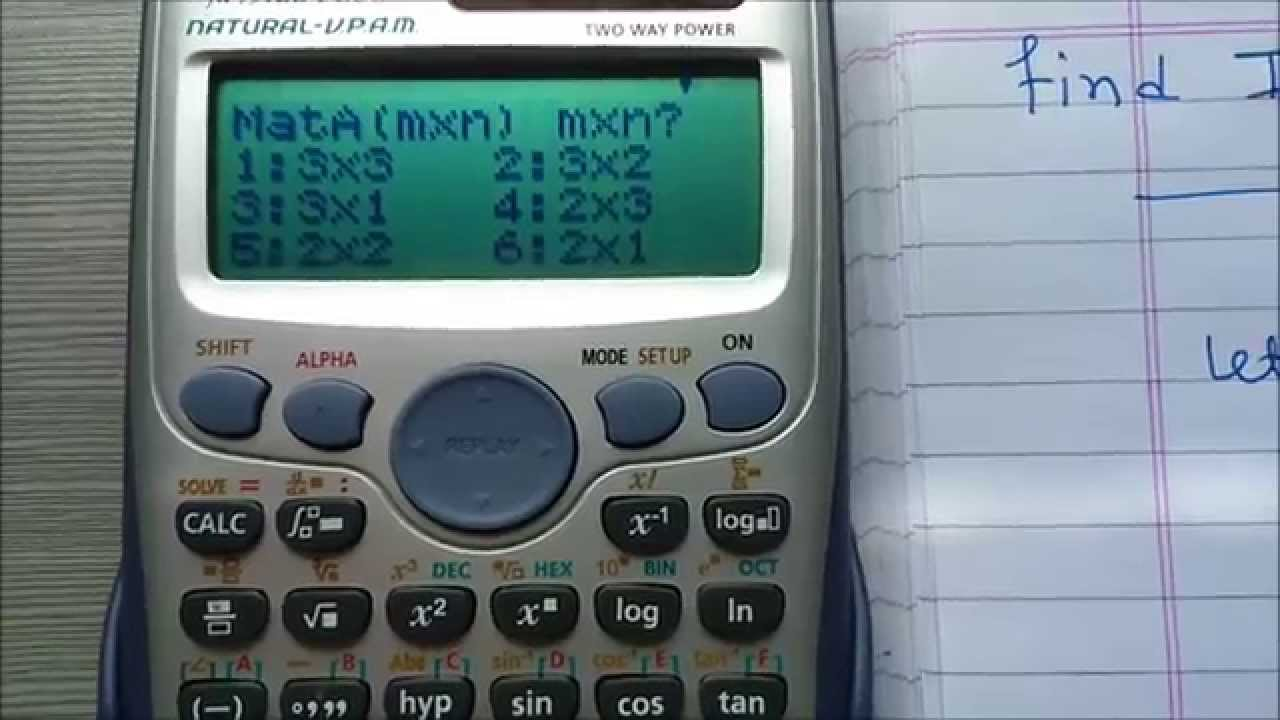5 – Calculating matrices

The majority of scientific calculators today should have this capability. One of the most tiresome computations students learn in high school is matrix calculation. Knowing how to use the calculator for matrix problems will therefore enable you to avoid doing time-consuming computations. Additionally, by using a calculator to perform the matrix calculations instead of completing them manually, you can lessen the likelihood of making any thoughtless errors. The drawback of utilizing a matrix calculator is that if you enter the incorrect value for one of the matrices, your calculations for that matrix may be incorrect or impossible to solve. To prevent such errors, students must double-check the values they enter.

6 – Calculations with numerical systems

If you are a student doing advanced mathematics, you must learn about numerical methods, where you use a variety of techniques to solve a set of differential equations. The chapter’s numerical techniques take a long time to finish and solve. For the majority of pupils, it is a problem with a guaranteed score, but computations must be done carefully to avoid any thoughtless errors in between in order to receive a complete mark. Therefore, using a scientific calculator helps reduce the likelihood that you will make careless errors when performing the calculations.

7 – Solving multiple equations at once

Some of the math calculator abilities on this list are excellent for aiding pupils in answering test questions. Many high school pupils are accustomed to solving simultaneous equations. A small number of kids can also complete them in under five minutes. You can use some of your calculator’s features after finishing your paper to assist you to double-check your answers. A guide on how to do it is provided below:

## Related Posts

#### Pre-U & University## share with the world

[affiliate_conversion_script amount="15" description="Free Trial Pop Up" context="Contact Form" status="unpaid" type="lead"]